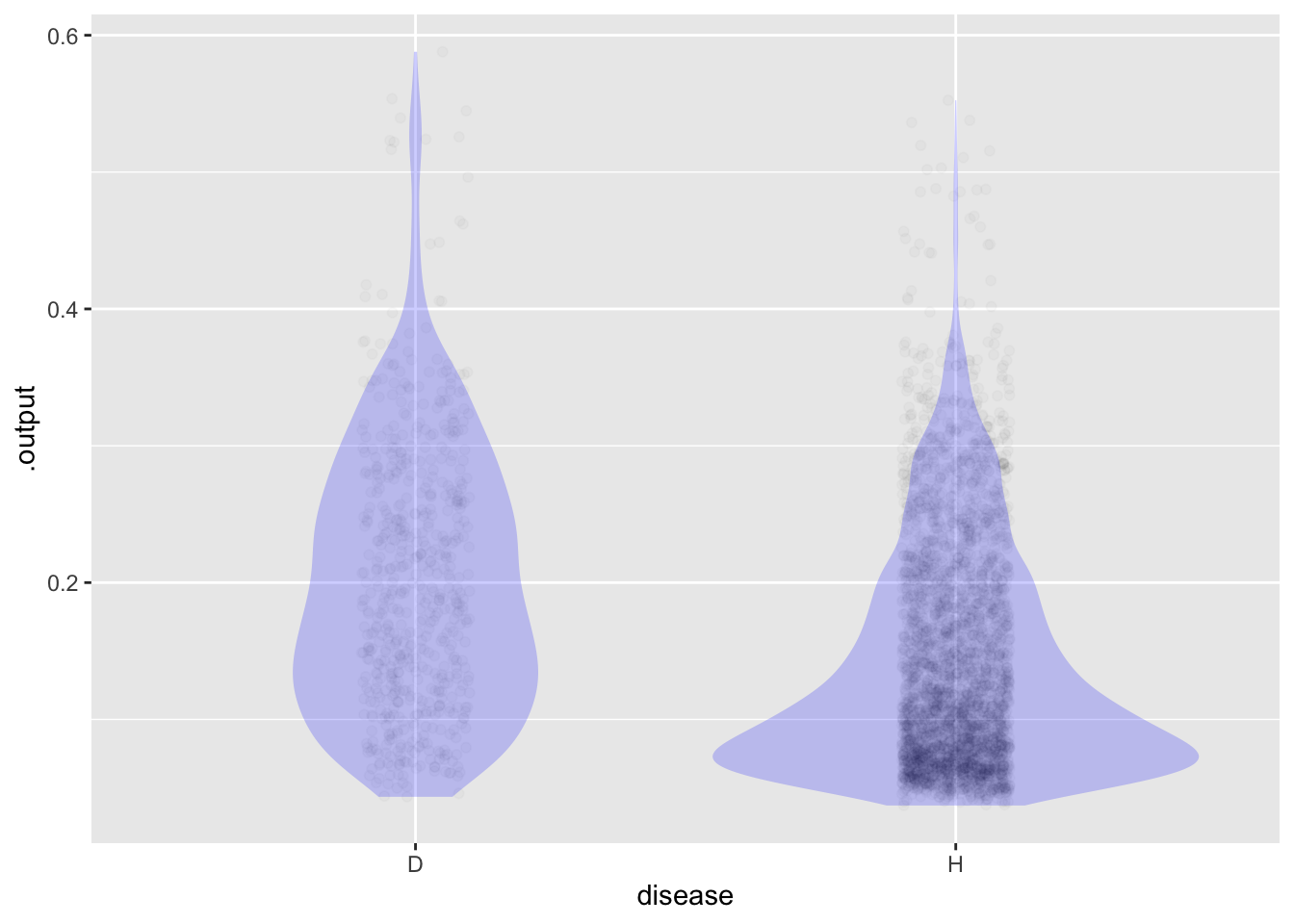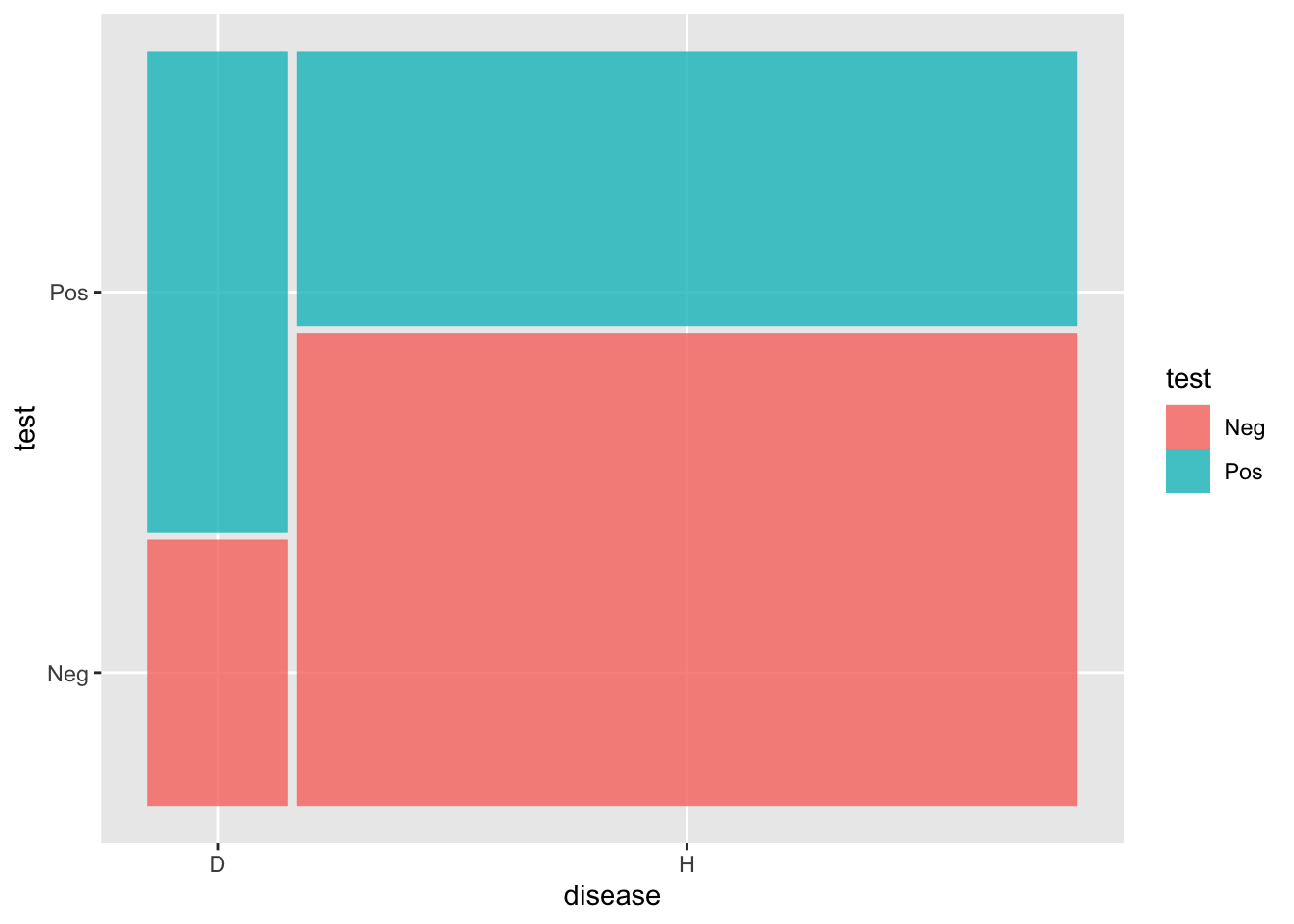# What do we want to get from teaching hypothesis testing?

## Summary

Putting hypothesis testing into a broader, more useful framework.

## Objectives for core statistics

1. Develop critical thinking and understanding of decision-making.
2. Empower students
1. technical mastery
2. confidence that they can think about data and evidence

Critical thinking: Ability to evaluate an argument (among other things)

Argument: Reasoning to demonstrate the truth/falsity/credibility of a hypothesis.

Hypothesis: A statement that might or might not be true. Examples:

1. We should treat you for … CHD or cancer or …
2. The object I’m tracking is a threat, so we should fire.

Conclusion from an argument: A statement about the truth/falsity or credibility of a hypothesis.

## Two forms of reasoning

I. Deductive reasoning: Reasoning from premise to conclusion (hypothesis truth/falsity/credibility) starting from premises/assumptions and using accepted mechanics of creating new statements from existing statements.

Deductive reasoning and critical thinking: Know how to “stress test” an argument. Two approaches: i. Check the mechanics for correctness. ii. Challenge the premises

II. Inductive reasoning: Reasoning from observations/evidence to conclusion. This is often called statistical inference.

Classical definition of statistical inference: “The process of using a sample to infer the properties of a population.” But this limited context has little or nothing to do with decision-making.

Methods of calculation:

1. Data from an observational study or experiment
2. Two settings
1. Confidence intervals: bootstrapping or probability algebra.
2. Hypothesis testing: Enforce Null hypotheses by permutation or probability algebra.

Classically strained definition of confidence intervals: What does the interval have to do with the population? It’s a statement about future, potential samples from the population: “In 95% of these future trials, the population parameter will be within the confidence interval.

Stat 101 Stress tests: check mechanics of calculation, check random sampling/random assignment. Awareness of sources of bias: survival, non-response, placebo, Hawthorne, …

## Broader framework for inductive reasoning

Based on an observation, compare and compete different hypotheses and the resulting decisions.

Simplification for Math 300: based on an observation compare two hypotheses and the resulting decision.

Initial context: Screening tests.

Examples of hypothesis pairs:

1. threat vs not a threat
2. disease vs no disease

We observe a test result and based on that prefer one of the hypotheses and the corresponding action.

Background: We collected lots of data (“training data”) where we know which hypothesis was correct and the output from the test.

Code
``cat("Training data")``
``Training data``
Code
``head(Framingham)``
``````# A tibble: 6 × 16
age education   curre…¹ cigsP…² BPMeds preva…³ preva…⁴ diabe…⁵ totChol sysBP
<dbl> <chr>       <chr>     <dbl> <chr>  <chr>   <chr>   <chr>     <dbl> <dbl>
1    39 college_gr… nonsmo…       0 no     none    none    not         195  106
2    46 HS grad     nonsmo…       0 no     none    none    not         250  121
3    48 some HS     smoker       20 no     none    none    not         245  128.
4    61 some colle… smoker       30 no     none    high BP not         225  150
5    46 some colle… smoker       23 no     none    none    not         285  130
6    43 HS grad     nonsmo…       0 no     none    high BP not         228  180
# … with 6 more variables: diaBP <dbl>, BMI <dbl>, heartRate <dbl>,
#   glucose <dbl>, TenYearCHD <dbl>, sex <chr>, and abbreviated variable names
#   ¹​currentSmoker, ²​cigsPerDay, ³​prevalentStroke, ⁴​prevalentHyp, ⁵​diabetes``````
Code
``cat("Model")``
``Model``
Code
``````mod <- glm(TenYearCHD ~ age + diabetes + totChol,
data=Framingham, family=binomial)
Scores <- model_eval(mod, interval="none") |>
mutate(disease=ifelse(.response == 1, "D", "H"))``````
``Using training data as input to model_eval().``
Code
``cat("Scores from training data.\n")``
``Scores from training data.``
Code
``head(Scores)``
``````  .response age diabetes totChol    .output      .resid disease
1         0  39      not     195 0.06008126 -0.06008126       H
2         0  46      not     250 0.10538177 -0.10538177       H
3         0  48      not     245 0.11906918 -0.11906918       H
4         1  61      not     225 0.25257802  0.74742198       D
5         0  46      not     285 0.11144294 -0.11144294       H
6         0  43      not     228 0.08332748 -0.08332748       H``````
Code
``cat("Picking a threshold and counting\n")``
``Picking a threshold and counting``
Code
``````my_threshold <- 0.15
Scores <- Scores |>
mutate(test = ifelse(.output > my_threshold, "Pos", "Neg"))
Counts <- Scores |>
group_by(disease, test) |>
tally()

ggplot(Scores, aes(x=disease, y=.output)) +
geom_jitter(alpha=.02, width=.1, height=0) +
geom_violin(alpha=.2, color=NA, fill="blue")``````Code
``cat("Apply threshold and count")``
``Apply threshold and count``
Code
``````my_threshold <- 0.15
Counts``````
``````# A tibble: 4 × 3
# Groups:   disease 
disease test      n
<chr>   <chr> <int>
1 D       Neg     226
2 D       Pos     409
3 H       Neg    2247
4 H       Pos    1306``````
Code
``````# in "wide" data frame
Counts |>
tidyr::pivot_wider(names_from=test, values_from=n)``````
``````# A tibble: 2 × 3
# Groups:   disease 
disease   Neg   Pos
<chr>   <int> <int>
1 D         226   409
2 H        2247  1306``````
Code
``````# as the canonical table
Iconic_graph <- Scores |>
ggplot() +
geom_mosaic(aes(x=product(disease), fill=test))
Iconic_graph``````
``````Warning: `unite_()` was deprecated in tidyr 1.2.0.
ℹ The deprecated feature was likely used in the ggmosaic package.
Please report the issue at <]8;;https://github.com/haleyjeppson/ggmosaichttps://github.com/haleyjeppson/ggmosaic]8;;>.``````### Apply loss function to evaluate threshold

Code
``````Counts\$cost = c(10,0,0,1)
Counts |> ungroup() |> summarize(total_cost = sum(n*cost))``````
``````# A tibble: 1 × 1
total_cost
<dbl>
1       3566``````

## False-positives, false-negatives, etc.

Sensitivity, specificity, and prevalence

Code
``````Counts |> group_by(disease) |>
mutate(prob = n/sum(n))``````
``````# A tibble: 4 × 5
# Groups:   disease 
disease test      n  cost  prob
<chr>   <chr> <int> <dbl> <dbl>
1 D       Neg     226    10 0.356
2 D       Pos     409     0 0.644
3 H       Neg    2247     0 0.632
4 H       Pos    1306     1 0.368``````

## Calculations using iconic graph